search
Search
Unlock 100+ guides
search toc
close
account_circle
Profile
exit_to_app
Sign out
search
keyboard_voice
close
Searching Tips
Search for a recipe:
"Creating a table in MySQL"
Search for an API documentation: "@append"
Search for code: "!dataframe"
Apply a tag filter: "#python"
Useful Shortcuts
/ to open search panel
Esc to close search panel
to navigate between search results
d to clear all current filters
Enter to expand content previewDoc SearchCode Search BetaSORRY NOTHING FOUND!
mic
Start speaking...Voice search is only supported in Safari and Chrome.
Shrink
Navigate to
check_circle
Mark as learned
thumb_up
4
thumb_down
0
chat_bubble_outline
0
Comment
auto_stories Bi-column layout
settings

# Comprehensive Guide to RDD in PySpark

schedule Aug 12, 2023
Last updated
local_offer
PySpark
Tags
mode_heat
Master the mathematics behind data science with 100+ top-tier guides
Start your free 7-days trial now!

# Prerequisites

You should already be familiar with the basics of PySpark. For a refresher, check out our Getting Started with PySpark guide.

# What is a RDD?

PySpark operates on big data by partitioning the data into smaller subsets spread across multiple machines. This allows for parallelisation, and this is precisely why PySpark can handle computations on big data efficiently. Under the hood, PySpark uses a unique data structure called RDD, which stands for resilient distributed dataset. In essence, RDD is an immutable data structure in which the data is partitioned across a number of worker nodes to facilitate parallel operations: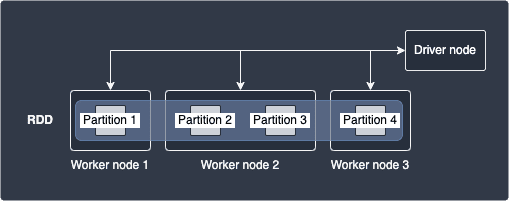In the diagram above, a single RDD has 4 partitions that is distributed across 3 worker nodes with the second worker node holding 2 partitions. By definition, a single partition cannot span across multiple worker nodes. This means, for instance, that partition 2 can never partially reside in both worker node 1 and 2 - the partition can only reside in either of worker node 1 or 2. The Driver node serves to coordinate the task execution between these worker nodes.

# Transformations and actions

There are two operations we can perform on a RDD:

• Transformations

• Actions

## Transformations

Transformations are basically functions applied on RDDs, which result in the creation of new RDDs. RDDs are immutable, which means that even after applying a transformation, the original RDD is kept intact. Examples of transformations include `map(~)` and `filter(~)`.

For instance, consider the following RDD transformation: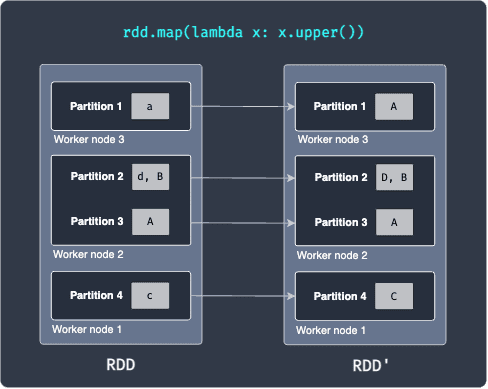Here, our `RDD` has 4 partitions that are distributed across 3 worker nodes. Partition 1 holds the string a, partition 2 holds the values `[d,B]` and so on. Suppose we now apply a map transformation that converts the string into uppercase. After the running the map transformation, we end up with `RDD'` shown on the right. What's important here is that each worker node performs the map transformation on the data it possesses - this is what makes distributed computing so efficient!

Since transformations return a new RDD, we can keep on applying transformations. The following example shows the creation of two new RDDs after applying two separate transformations: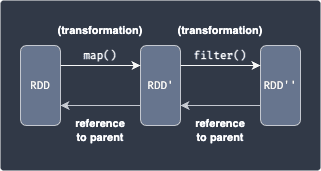Here, we apply the `map(~)` transformation to a `RDD`, which applies a function to each data in `RDD` to yield `RDD'`. Next, we apply the `filter(~)` transformation to select a subset of the data in `RDD'` to finally obtain `RDD''`.

Spark keeps track of the series of transformations applied to RDD using graphs called RDD lineage or RDD dependency graphs. In the above diagram, `RDD` is considered to be a parent of `RDD'`. Every child RDD has a reference to its parent (e.g. `RDD'` will always have a reference to `RDD`).

## Actions

Actions are operations that either:

• send all the data held by multiple nodes to the driver node. For instance, printing some result in the driver node (e.g. `show(~)`).

• or saving some data on an external storage system such as HDFS and Amazon S3. (e.g. `saveAsTextFile(~)`).

Typically, actions are followed by a series of transformations like so: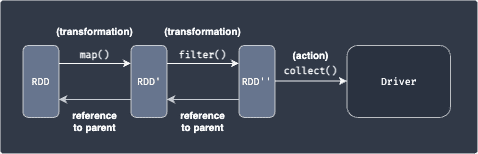After applying transformations, the actual data of the output RDD still reside in different nodes. Actions are used to gather these scattered results in a single place - either the driver node or an external data storage.

NOTE

Transformations are lazy, which means that even if you call the `map(~)` function, Spark will not actually do anything behind the scenes. All transformations are only executed once an action, such as `collect(~)`, is triggered. This allows Spark to optimise the transformations by:

• allocating resource more efficiently

• grouping transformations together to avoid network traffic

## Example using PySpark

Consider the same set of transformations and action from earlier:Here, we are first converting each string into uppercase using the transformation `map(~)`, and then performing a `filter(~)` transformation to obtain a subset of the data. Finally, we send the individual results held in different partitions to the driver node to print the final result on the screen using the action `show()`.

Consider the following RDD with 3 partitions:

``` rdd = sc.parallelize(["Alex","Bob","Cathy"], numSlices=3)rdd.collect() ['Alex', 'Bob', 'Cathy'] ```

Here:

• `sc`, which stands for SparkContext, is a global variable defined by Databricks.

• we are using the `parallelize(~)` method of SparkContext to create a RDD.

• the number of partitions is specified using the `numSlices` argument.

• the `collect(~)` method is used to gather all the data from each partition to the driver node and print the results on the screen.

Next, we use the `map(~)` transformation to convert each string (which resides in different partitions) to uppercase. We then use the `filter(~)` transformation to obtain strings that equal `"ALEX"`:

``` rdd2 = rdd1.map(lambda x: x.upper())rdd3 = rdd2.filter(lambda name: name == "ALEX")rdd3.collect() ['ALEX'] ```
NOTE

To run this example, visit our guide Getting Started with PySpark on Databricks.

# Narrow and wide transformations

There are two types of transformations:

• Narrow - no shuffling is needed, which means that data residing in different nodes do not have to be transferred to other nodes

• Wide - shuffling is required, and so wide transformations are costly

The difference is illustrated below: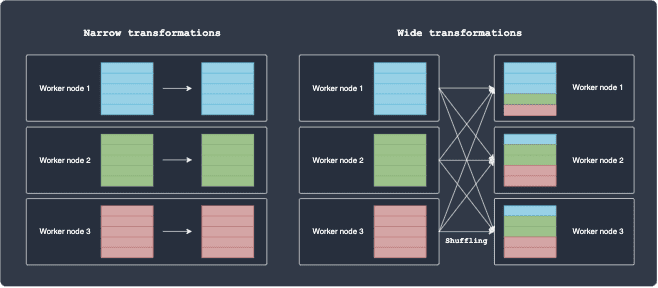For narrow transformations, the partition remains in the same node after the transformation, that is, the computation is local. In contrast, wide transformations involve shuffling, which is slow and expensive because of network latency and bandwidth.

Some examples of narrow transformations include `map(~)` and `filter(~)`. Consider a simple map operation where we increment an integer of some data by one. It's clear that the each worker node can perform this on their own since there is no dependency between the partitions living on other worker nodes.

Some examples of wide transformations include `groupBy(~)` and `sort(~)`. Suppose we wanted to perform a `groupBy(~)` operation on some column, say a categorical variable consisting of 3 classes: `A`, `B` and `C`. The following diagram illustrates how Spark will execute this operation: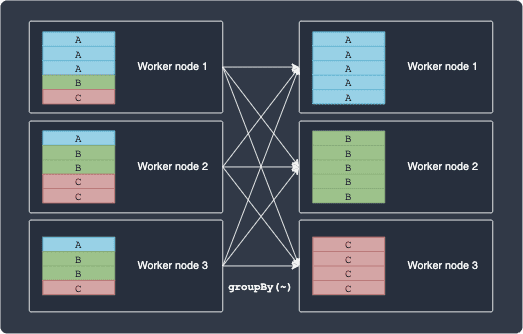Notice how `groupBy(~)` cannot be computed locally because the operation requires dependency between partitions lying in different nodes.

# Fault tolerance property

The R in RDD stands for resilient, meaning that even if a worker node fails, the missing partition can still be recomputed to recover the RDD with the help of RDD lineage. For instance, consider the following example:Suppose `RDD''` is "damaged" because of a node failure. Since Spark knows that `RDD'` is the parent of `RDD''`, Spark will be able to re-compute `RDD''` from `RDD'`.

# Viewing the underlying partitions of a RDD in PySpark

Let's create a RDD in PySpark by using the `parallelize(~)` method once again:

``` rdd = sc.parallelize(["a","B","c","D"])rdd.collect() ['a', 'B', 'c', 'D'] ```

To see the underlying partition of the RDD, use the `glom()` method like so:

``` rdd.glom().collect() [[], ['a'], [], ['B'], [], ['c'], [], ['D']] ```

Here, we see that the RDD has 8 partitions by default. This default number of partitions can be set in the Spark configuration file. Because our RDD only contains 4 values, we see that half of the partitions are empty.

We can specify that we want to break down our data into say `3` partitions by supplying the `numSlices` parameter:

``` rdd = sc.parallelize(["a","B","c","D"], numSlices=3)rdd.glom().collect() [['a'], ['B'], ['c', 'D']] ```

# Difference between RDD and DataFrames

When working with PySpark, we usually use DataFrames instead of RDDs. Similar to RDDs, DataFrames are also an immutable collection of data, but the key difference is that DataFrames can be thought of as a spreadsheet-like table where the data is organised into columns. This does limit the use-case of DataFrames to only structured or tabular data, but the added benefit is that we can work with our data at a much higher level of abstraction. If you've ever used a Pandas DataFrame, you'll understand just how easy it is to interact with your data.

DataFrames are actually built on top of RDDs, but there are still cases when you would rather work at a lower level and tinker directly with RDDs. For instance, if you are dealing with unstructured data (e.g. audio and streams of data), you would use RDDs rather than DataFrames.

NOTE

If you are dealing with structured data, we highly recommend that you use DataFrames instead of RDDs. This is because Spark will optimize the series of operations you perform on DataFrames under the hood, but will not do so in the case of RDDs.

## Seeing the partitions of a DataFrame

Since DataFrames are built on top of RDDs, we can easily see the underlying RDD representation of a DataFrame. Let's start by creating a simple DataFrame:

``` columns = ["Name", "Age"]df = spark.createDataFrame([["Alex", 15], ["Bob", 20], ["Cathy", 30]], columns)df.show() +-----+-----+---+| Name|Group|Age|+-----+-----+---+| Alex| A| 15|| Bob| A| 20||Cathy| A| 30|+-----+-----+---+ ```

To see how this DataFrame is partitioned by its underlying RDD:

``` df.rdd.glom().collect() [[], [], [Row(Name='Alex', Age=15)], [], [], [Row(Name='Bob', Age=20)], [], [Row(Name='Cathy', Age=30)]] ```

We see that our DataFrame is partitioned in terms of `Row`, which is a native object in PySpark.

thumb_up
thumb_down
Comment
Citation
Ask a question or leave a feedback...
thumb_up
4
thumb_down
0
chat_bubble_outline
0
settings
Enjoy our search
Hit / to insta-search docs and recipes!